Algebra

# Translating Graphs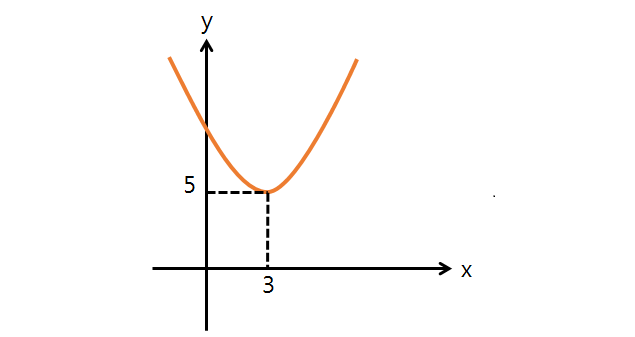The above graph is the result of parallel translation of $y = x^2.$ If this graph undergoes an additional translation of $5$ in the positive direction of the $x$-axis and an additional translation of $6$ in the positive direction of the $y$-axis, what is the equation of the resulting graph?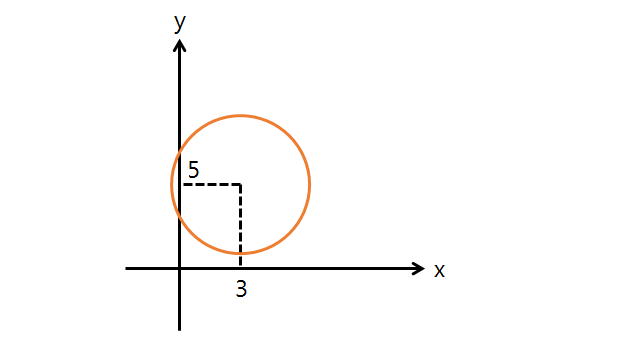If the graph of the above circle with center $(3,5)$ and radius $4$ undergoes a translation of $4$ in the positive direction of the $x$-axis and $5$ in the positive direction of the $y$ axis, what is the equation of the resulting graph in quadratic form?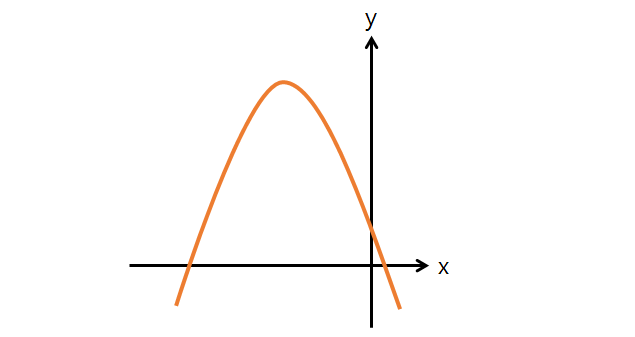If the graph of $y = -8 x^2 -80 x -38$ undergoes a translation of $-3$ in the positive direction of the $x$-axis and $3$ in the positive direction of the $y$-axis, what is the equation of the resulting graph?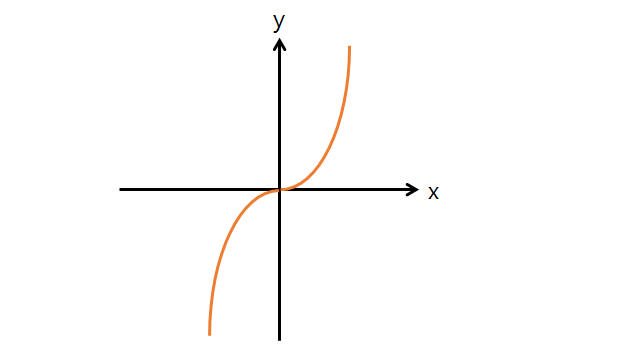If the above graph of $y = 9 x^3$ is the result of a parallel translation of the equation $y=f(x)$ by $- 5$ in the positive direction of the $x$-axis followed by a translation of $- 3$ in the positive direction of the $y$-axis, what is the equation $y=f(x)?$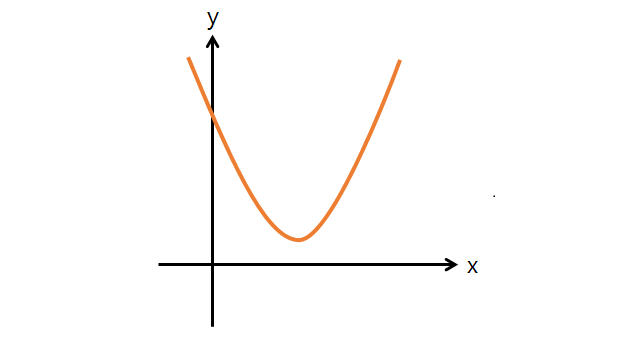If the graph of $y = 5 (x-5)^2 + 7$ undergoes a translation of $3$ in the positive direction of the $x$ axis and $-1$ in the positive direction of the $y$-axis, what is the equation of the resulting graph?

×